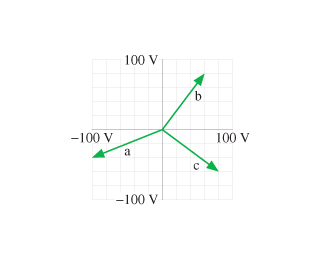# Problem: The figure shows emf phasors a, b, and c.Part AFor each, what is the instantaneous value of the emf?Part BAt this instant, is the magnitude of emf represented by phasor a increasing, decreasing, or holding constant?

###### FREE Expert Solution

The instantaneous emf is expressed as:

$\overline{){\mathbf{V}}{\mathbf{=}}{{\mathbf{V}}}_{{\mathbf{0}}}{\mathbf{c}}{\mathbf{o}}{\mathbf{s}}{\mathbf{\left(}}{\mathbf{\omega }}{\mathbf{t}}{\mathbf{+}}{\mathbf{\varphi }}{\mathbf{\right)}}}$

Part A

The instantaneous value of the emf is the projection of the phasor on the horizontal axis.

92% (72 ratings)###### Problem Details

The figure shows emf phasors a, b, and c.Part A

For each, what is the instantaneous value of the emf?

Part B

At this instant, is the magnitude of emf represented by phasor a increasing, decreasing, or holding constant?